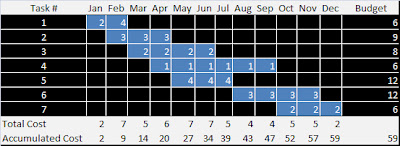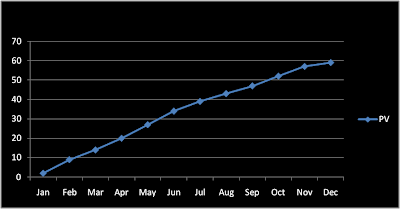### Planned Value

The Planned Value (PV) is a function of the time and It represents the economic value that will be spent in a project. The domain is time and the range is economic value (\$). It associates a value to each moment of time. It's a convention that the Planned Value (PV) starts at the time zero and goes until the estimated duration of the project. The minimun value is zero and the maximun value of the Planned Value (PV) is the total budget of the project.

The Planned Value (PV) is a consequence of sum the cost of the tasks of a project at the moment when these tasks need to be executed. As you can see in the following table, the Planned Value (PV) is the accumulated cost of the execution of a project in the time.Clik to maximize
The curve obtained of the accumulated cost is the Planned Value (PV), also known as "Baseline" and is the reference for the cost performance for the whole project.Click to maximize